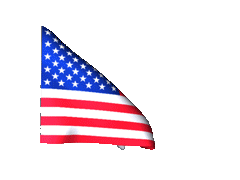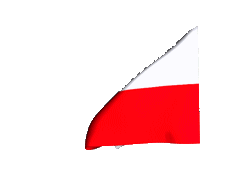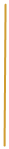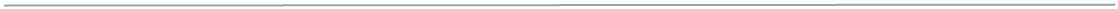College
Calculus Curriculum

1) Functions, Graphs, and Limits
A. Analysis of graphs;
B. Limits of functions , including one side limit;
C. Asymptotic and unbounded behavior;
D. Continuity as a property of functions.
2) Derivatives
A. Concept of the derivative via difference quotients;
B. Derivative at a point;
C. Derivative as a function,
D. Second and higher derivatives;
E. Applications of derivatives;
F. Computation of derivatives;
G. Function testing process variability.
3) Integrals
A. Interpretations and properties of definite Integrals;
B. Applications of integrals;
C. Fundamental theorem of calculus;
D. Improper integrals;
E. Techniques of antidifferentiation;
F. Applications of antidifferentiation;
G. Numerical approximations to definite integrals;
H. Using integrals to solve surface area and volume of figures limited by
curves -  multiple integration.